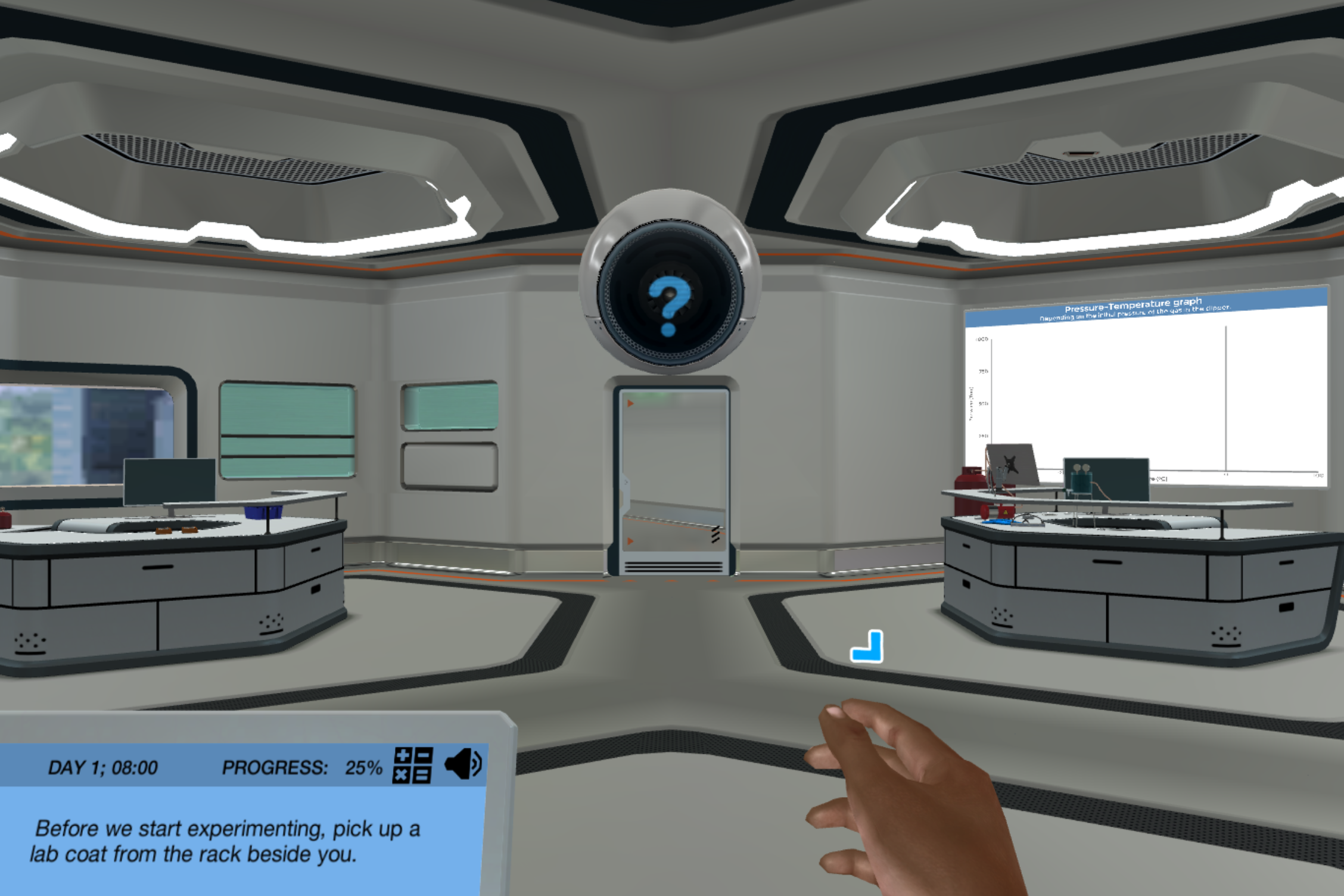# Ideal Gas Law: Introduction Virtual Lab

Observe the behavior of an ideal gas and the effects on this behavior when external variables are altered. Learn how the different variables affect the behavior and understand the relation of these variables to the ideal gas law equation.Try our lab safety simulation

Discover one of our 200+ learning simulations today

• Description
• Features

## About the Ideal Gas Law: Introduction Virtual Lab

This short, targeted simulation is adapted from the full-length “Ideal Gas Law” simulation.

Are you interested in seeing virtual ideal gas molecules? In this simulation, you will observe how ideal gas molecules behave according to the Ideal Gas Law. You’ll learn about the relationship between pressure, volume and temperature in gases by adjusting these variables. Unlike in a normal lab, you’ll be able to observe a physical representation of the gas molecules!

Formulate the Ideal Gas Law

Imagine how easy it would be to understand the behavior of an ideal gas if the gas molecules were so big that they could be seen with the naked eye. Well, in this simulation they can! The gas molecules are magnified, so you can see what is otherwise invisible. Your next mission is to define the relationship between the pressure, temperature and volume of an ideal gas sealed in a glass jar.

Familiarize yourself with the ideal gas law equation

Take a deeper look into the makeup of the ideal gas law equation and how each variable is directly correlated with one another. Relate the effect of the variables from observing the virtual gas molecules in the jar to the ideal gas law equation to understand that it defines the exact behavior you have been observing.

Consolidate all of your knowledge gained from observing the ideal gas molecules by answering some questions and reading up on relevant theory through our informative theory pages linked in the simulation. Become introduced to a method that could be used in a lab to collect data and prove that this is in fact how ideal gases behave.

Get started now

Observe the behavior of an ideal gas and the effects on this behavior when external variables are altered. Learn how the different variables affect the behavior and understand the relation of these variables to the ideal gas law equation.

Techniques in lab
Gas inlet, Bunsen burner, Ideal Gas Law
Learning objectives

At the end of this simulation, you will be able to...

• Define the relationship between pressure, volume and temperature in gases
• Relate the behavior of ideal gases to the ideal gas law equation.
Simulation features

Length: 15 Minutes
Accessibility mode: Available
Languages: English (United States)

NGSS

No direct alignment

IB

No direct alignment

AP

Chemistry Unit 3.4 Ideal gas law

Physics 2 Unit 2.2 Pressure, thermal Equilibrium, and the ideal gas law